# 题目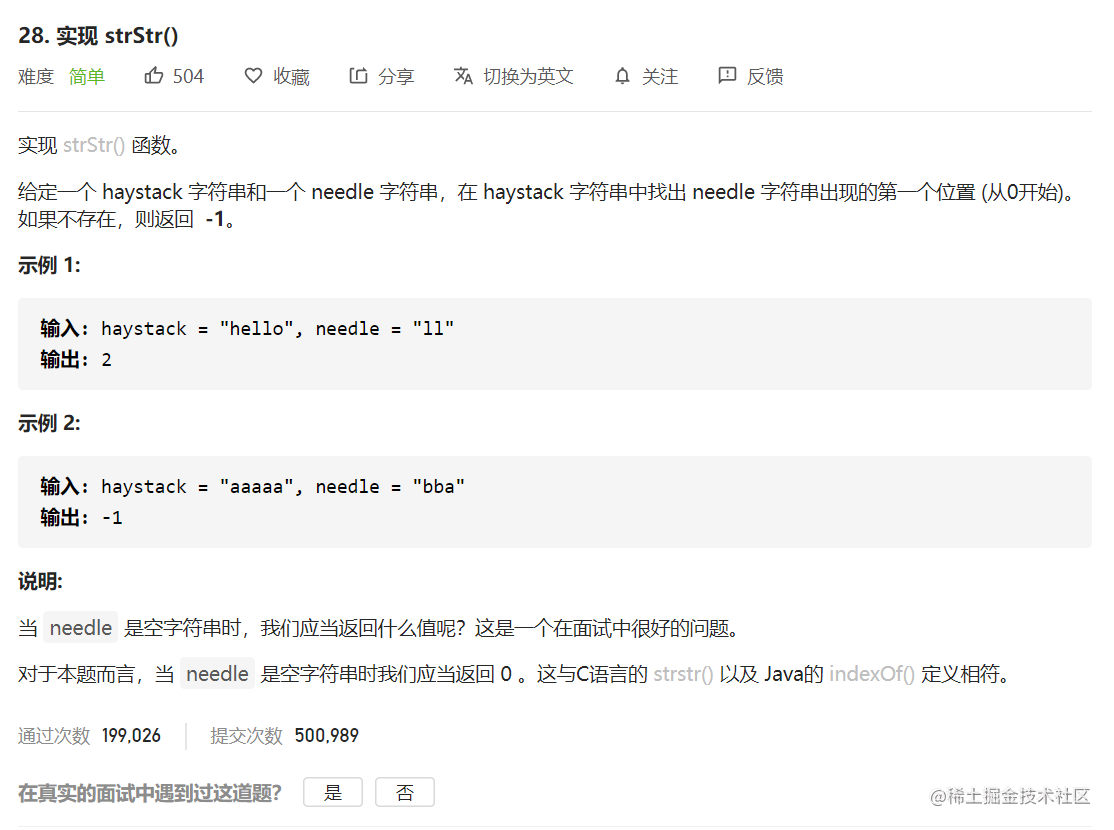# 分析

#### 概述

• Rabin-Karp，通过哈希算法实现常数时间窗口内字符串比较。
• 比特位操作，通过比特掩码来实现常数时间窗口内字符串比较。

#### 方法一：子串逐一比较 - 线性时间复杂度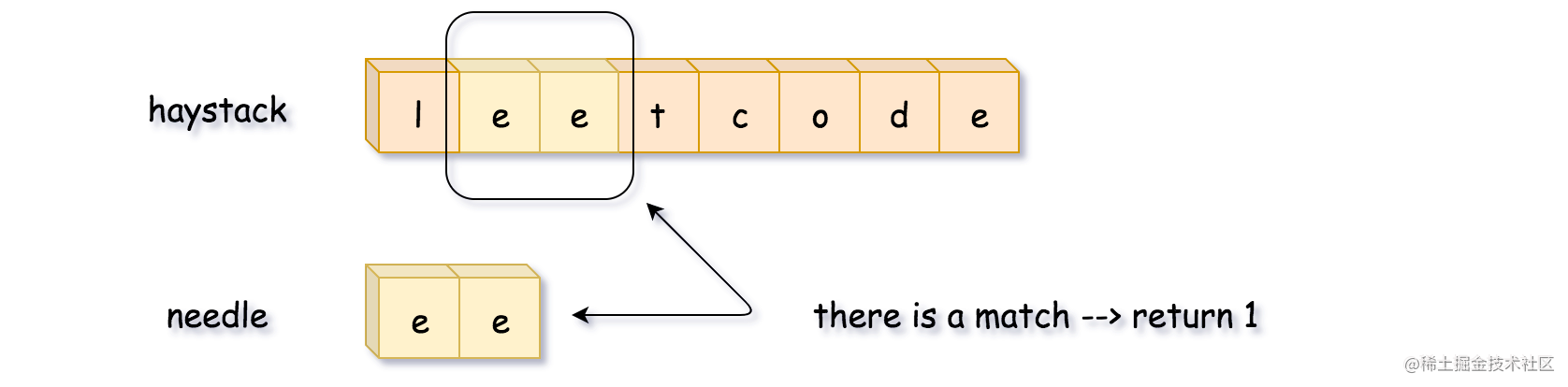class Solution {
public int strStr(String haystack, String needle) {
int L = needle.length(), n = haystack.length();

for (int start = 0; start < n - L + 1; ++start) {
if (haystack.substring(start, start + L).equals(needle)) {
return start;
}
}
return -1;
}
}

• 时间复杂度：O((N - L)L)，其中 N 为 haystack 字符串的长度，L 为 needle 字符串的长度。内循环中比较字符串的复杂度为 L，总共需要比较 (N - L) 次。
• 空间复杂度：O(1)。

#### 方法二：双指针 - 线性时间复杂度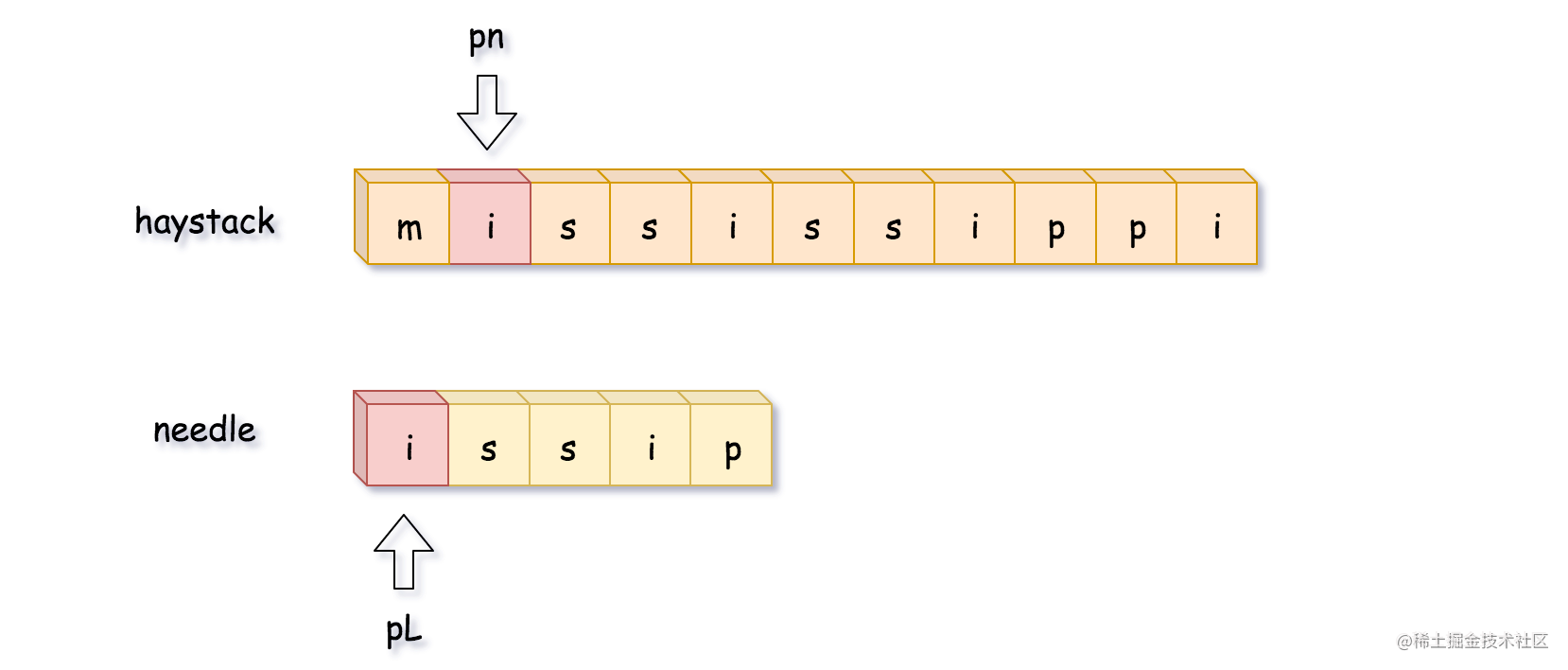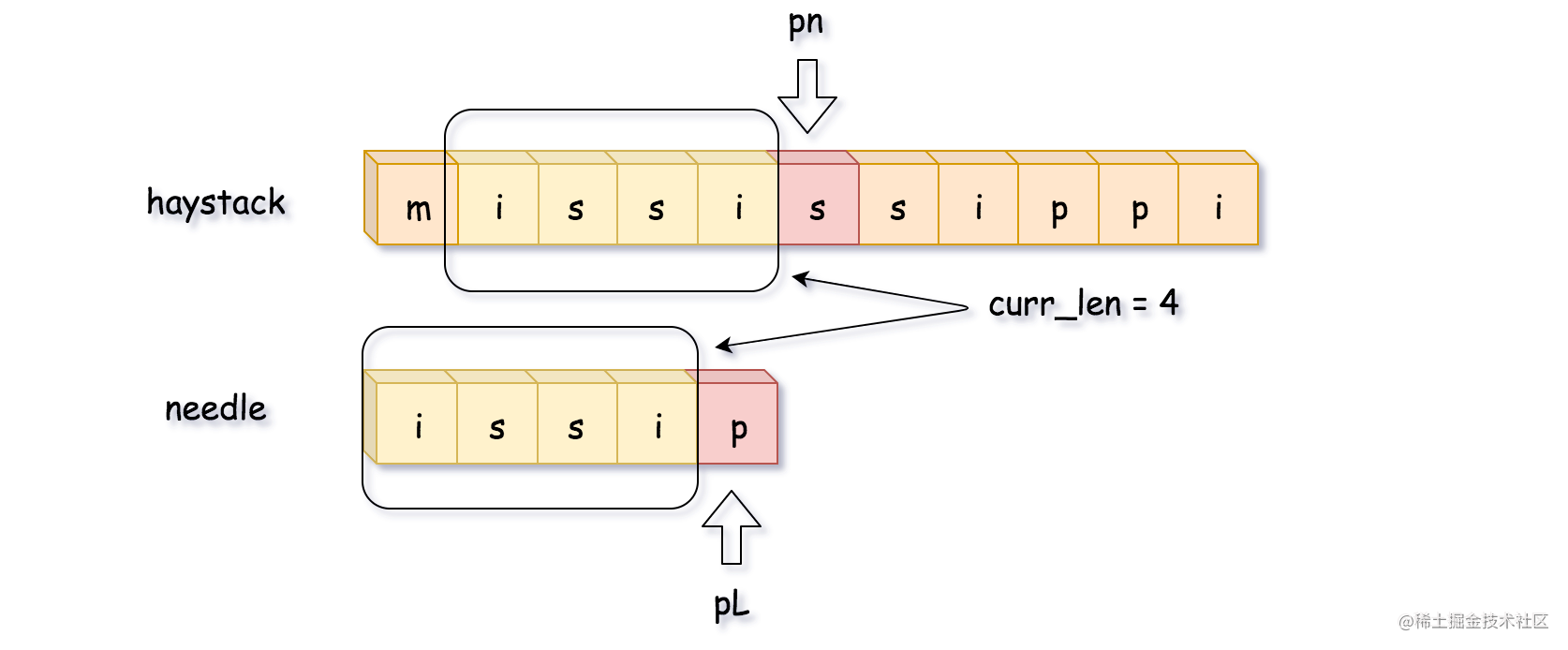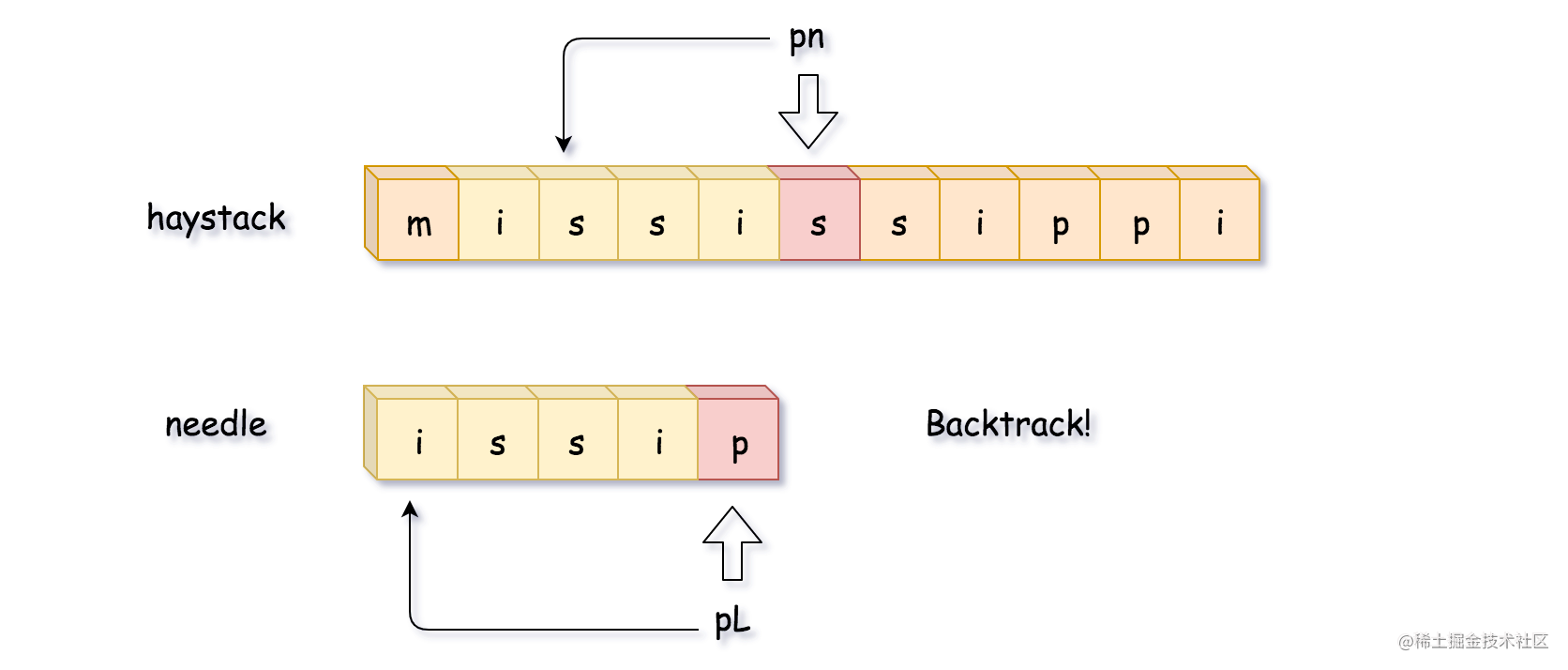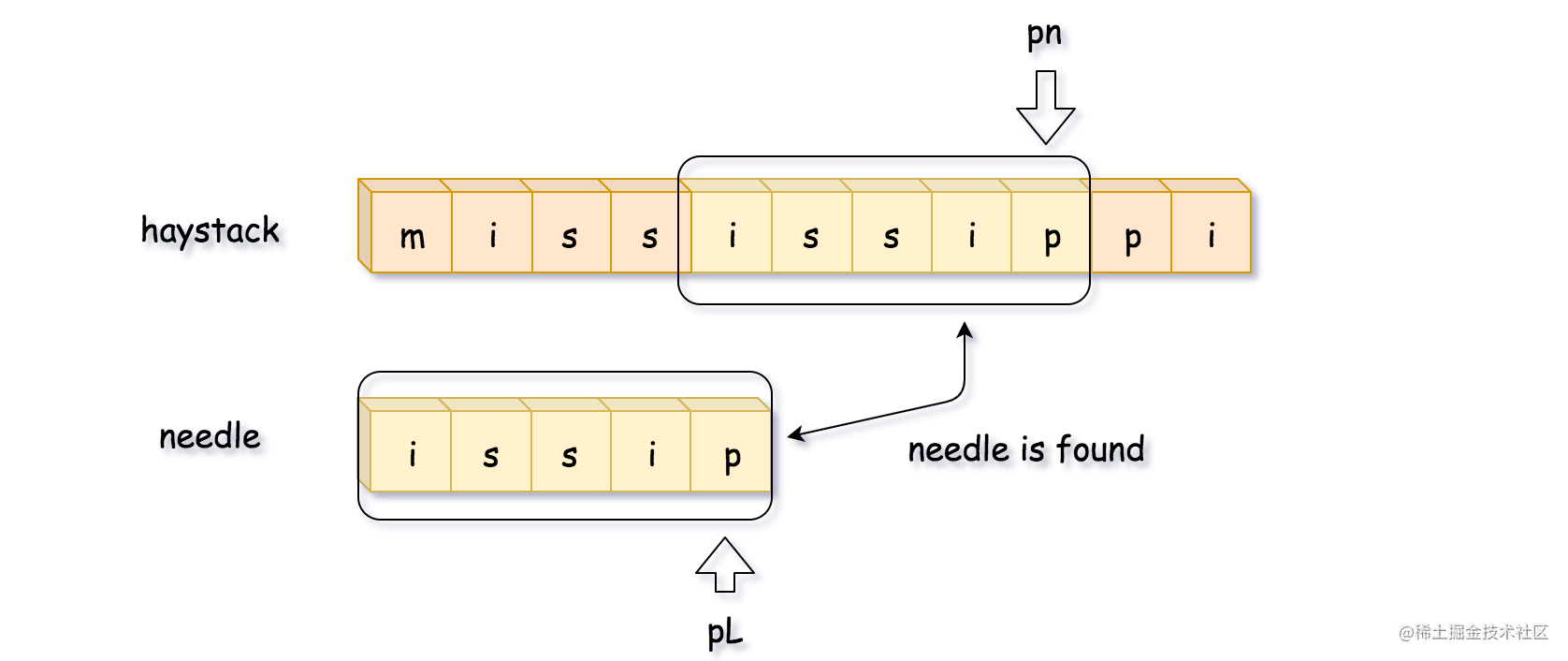• 移动 pn 指针，直到 pn 所指向位置的字符与 needle 字符串第一个字符相等。
• 通过 pn，pL，curr_len 计算匹配长度。
• 如果完全匹配（即 curr_len == L），返回匹配子串的起始坐标（即 pn - L）。
• 如果不完全匹配，回溯。使 pn = pn - curr_len + 1， pL = 0， curr_len = 0。

class Solution {
public int strStr(String haystack, String needle) {
int L = needle.length(), n = haystack.length();
if (L == 0) return 0;

int pn = 0;
while (pn < n - L + 1) {
// find the position of the first needle character
// in the haystack string
while (pn < n - L + 1 && haystack.charAt(pn) != needle.charAt(0)) ++pn;

// compute the max match string
int currLen = 0, pL = 0;
while (pL < L && pn < n && haystack.charAt(pn) == needle.charAt(pL)) {
++pn;
++pL;
++currLen;
}

// if the whole needle string is found,
// return its start position
if (currLen == L) return pn - L;

// otherwise, backtrack
pn = pn - currLen + 1;
}
return -1;
}
}

• 时间复杂度：最坏时间复杂度为 O((N - L)L)，最优时间复杂度为 O(N)。
• 空间复杂度：O(1)。

#### 方法三： Rabin Karp - 常数复杂度

h 0 = 0 × 2 6 3 + 1 × 2 6 2 + 2 × 2 6 1 + 3 × 2 6 0 h_0 = 0 \times 26^3 + 1 \times 26^2 + 2 \times 26^1 + 3 \times 26^0 h0​=0×263+1×262+2×261+3×260

h 0 = c 0 a L − 1 + c 1 a L − 2 + . . . + c i a L − 1 − i + . . . + c L − 1 a 1 + c L a 0 0 h_0 = c_0 a^{L - 1} + c_1 a^{L - 2} + ... + c_i a^{L - 1 - i} + ... + c_{L - 1} a^1 + c_L a^00 h0​=c0​aL−1+c1​aL−2+...+ci​aL−1−i+...+cL−1​a1+cL​a00

h 0 = ∑ i = 0 L − 1 c i a L − 1 − i h_0 = \sum_{i = 0}^{L - 1}{c_i a^{L - 1 - i}} h0​=∑i=0L−1​ci​aL−1−i

h 1 = ( h 0 − 0 × 2 6 3 ) × 26 + 4 × 2 6 0 h_1 = (h_0 - 0 \times 26^3) \times 26 + 4 \times 26^0 h1​=(h0​−0×263)×26+4×260

h 1 = ( h 0 a − c 0 a L ) + c L + 1 h_1 = (h_0 a - c_0 a^L) + c_{L + 1} h1​=(h0​a−c0​aL)+cL+1​

a^La**L 可能是一个很大的数字，因此需要设置数值上限来避免溢出。设置数值上限可以用取模的方式，即用 h % modulus 来代替原本的哈希值。

• 计算子字符串 haystack.substring(0, L)needle.substring(0, L) 的哈希值。

• 从起始位置开始遍历：从第一个字符遍历到第 N - L 个字符。

• 根据前一个哈希值计算滚动哈希。
• 如果子字符串哈希值与 needle 字符串哈希值相等，返回滑动窗口起始位置。
• 返回 -1，这时候 haystack 字符串中不存在 needle 字符串。

class Solution {
// function to convert character to integer
public int charToInt(int idx, String s) {
return (int)s.charAt(idx) - (int)'a';
}

public int strStr(String haystack, String needle) {
int L = needle.length(), n = haystack.length();
if (L > n) return -1;

// base value for the rolling hash function
int a = 26;
// modulus value for the rolling hash function to avoid overflow
long modulus = (long)Math.pow(2, 31);

// compute the hash of strings haystack[:L], needle[:L]
long h = 0, ref_h = 0;
for (int i = 0; i < L; ++i) {
h = (h * a + charToInt(i, haystack)) % modulus;
ref_h = (ref_h * a + charToInt(i, needle)) % modulus;
}
if (h == ref_h) return 0;

// const value to be used often : a**L % modulus
long aL = 1;
for (int i = 1; i <= L; ++i) aL = (aL * a) % modulus;

for (int start = 1; start < n - L + 1; ++start) {
// compute rolling hash in O(1) time
h = (h * a - charToInt(start - 1, haystack) * aL
+ charToInt(start + L - 1, haystack)) % modulus;
if (h == ref_h) return start;
}
return -1;
}
}

• 时间复杂度：O(N)，计算 needle 字符串的哈希值需要 O(L)O(L) 时间，之后需要执行 (N - L)(NL) 次循环，每次循环的计算复杂度为常数。
• 空间复杂度：O(1)。

### 方法四：Sunday算法

#### 一、Sunday 匹配机制

• 目标字符串String
• 模式串 Pattern
• 当前查询索引 idx （初始为 0）
• 待匹配字符串 str_cut : String [ idx : idx + len(Pattern) ]

• 若匹配，则返回当前 idx

• 不匹配，则查看 待匹配字符串 的后一位字符 c

1. c存在于Pattern中，则 idx = idx + 偏移表[c]
2. 否则，idx = idx + len(pattern)

Repeat Loop 直到 idx + len(pattern) > len(String)

#### 二、偏移表

• a 的偏移位就是 len(pattern)-1 = 2
• b 的偏移位就是 len(pattern)-2 = 1
• 其他的均为 len(pattern)+1 = 4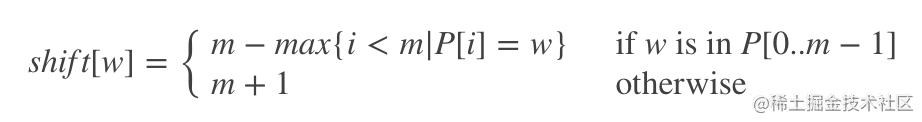#### 三、举例

String: checkthisout
Pattern: this

#### Step 1: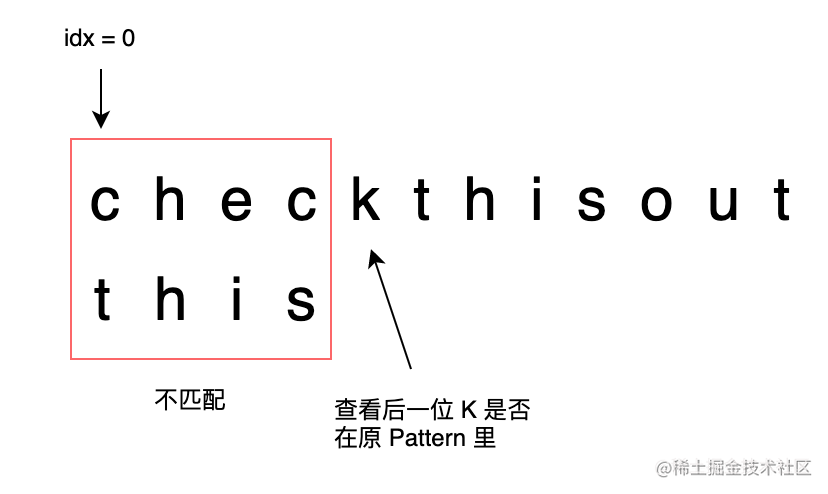• idx = 0
• 待匹配字符串为：chec
• 因为 chec != this
• 所以查看 chec 的下一个字符 k
• k 不在 Pattern 里
• 所以查看 偏移表，idx = idx + 5

#### Step 2: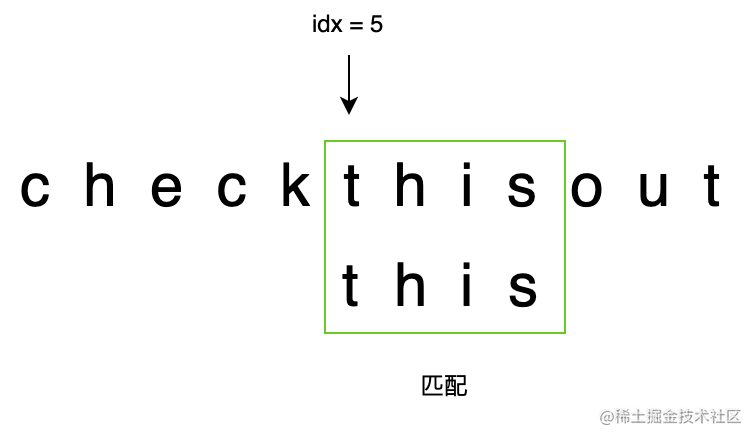• idx = 5
• 待匹配字符串为：this
• 因为 this == this
• 匹配，所以返回 5

#### 五、代码

class Solution:
def strStr(self, haystack: str, needle: str) -> int:

# Func: 计算偏移表
def calShiftMat(st):
dic = {}
for i in range(len(st)-1,-1,-1):
if not dic.get(st[i]):
dic[st[i]] = len(st)-i
dic["ot"] = len(st)+1
return dic

# 其他情况判断
if len(needle) > len(haystack):return -1
if needle=="": return 0

# 偏移表预处理
dic = calShiftMat(needle)
idx = 0

while idx+len(needle) <= len(haystack):

# 待匹配字符串
str_cut = haystack[idx:idx+len(needle)]

# 判断是否匹配
if str_cut==needle:
return idx
else:
# 边界处理
if idx+len(needle) >= len(haystack):
return -1
# 不匹配情况下，根据下一个字符的偏移，移动idx
cur_c = haystack[idx+len(needle)]
if dic.get(cur_c):
idx += dic[cur_c]
else:
idx += dic["ot"]

return -1 if idx+len(needle) >= len(haystack) else idx

### 方法五：KMP算法

KMP 算法（Knuth-Morris-Pratt 算法）是一个著名的字符串匹配算法，效率很高，但是确实有点复杂。

PS：本文的代码参考《算法4》，原代码使用的数组名称是 dfa（确定有限状态机），我对书中代码进行了一点修改，并沿用 dp 数组的名称。

### 一、KMP 算法概述

// 暴力匹配（伪码）
int search(String pat, String txt) {
int M = pat.length;
int N = txt.length;
for (int i = 0; i < N - M; i++) {
int j;
for (j = 0; j < M; j++) {
if (pat[j] != txt[i+j])
break;
}
// pat 全都匹配了
if (j == M) return i;
}
// txt 中不存在 pat 子串
return -1;
}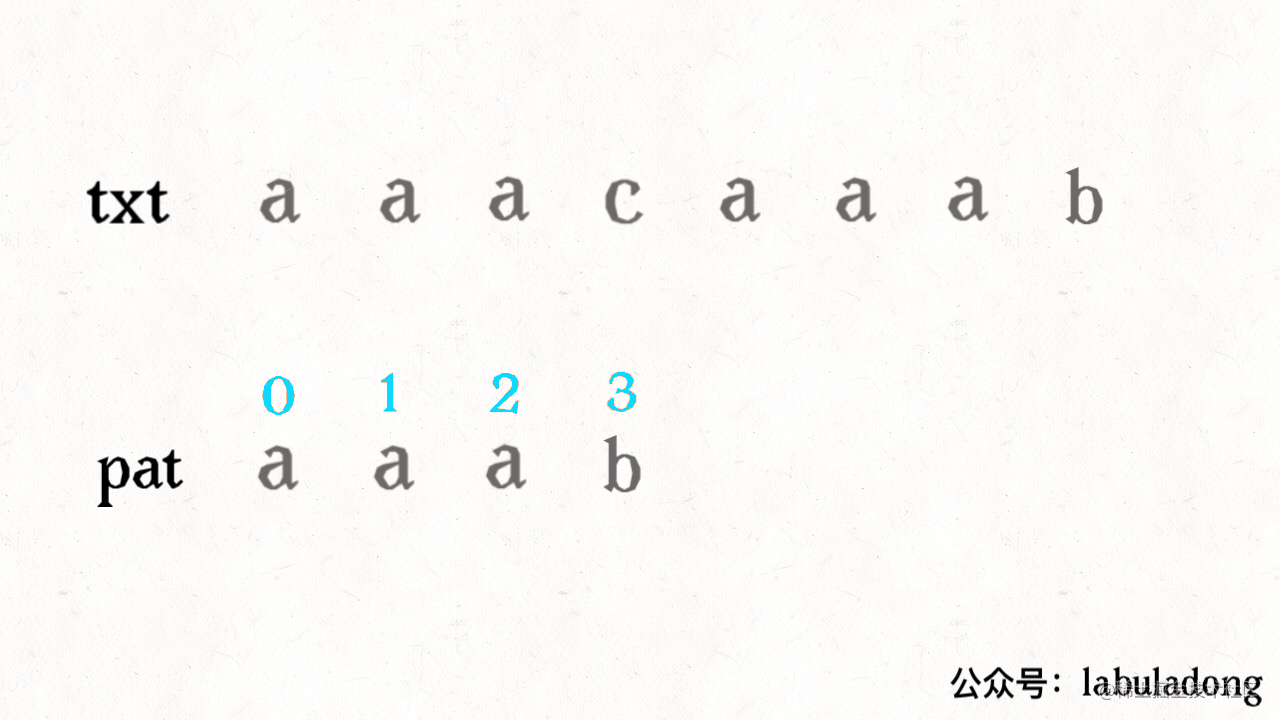KMP 算法的不同之处在于，它会花费空间来记录一些信息，在上述情况中就会显得很聪明：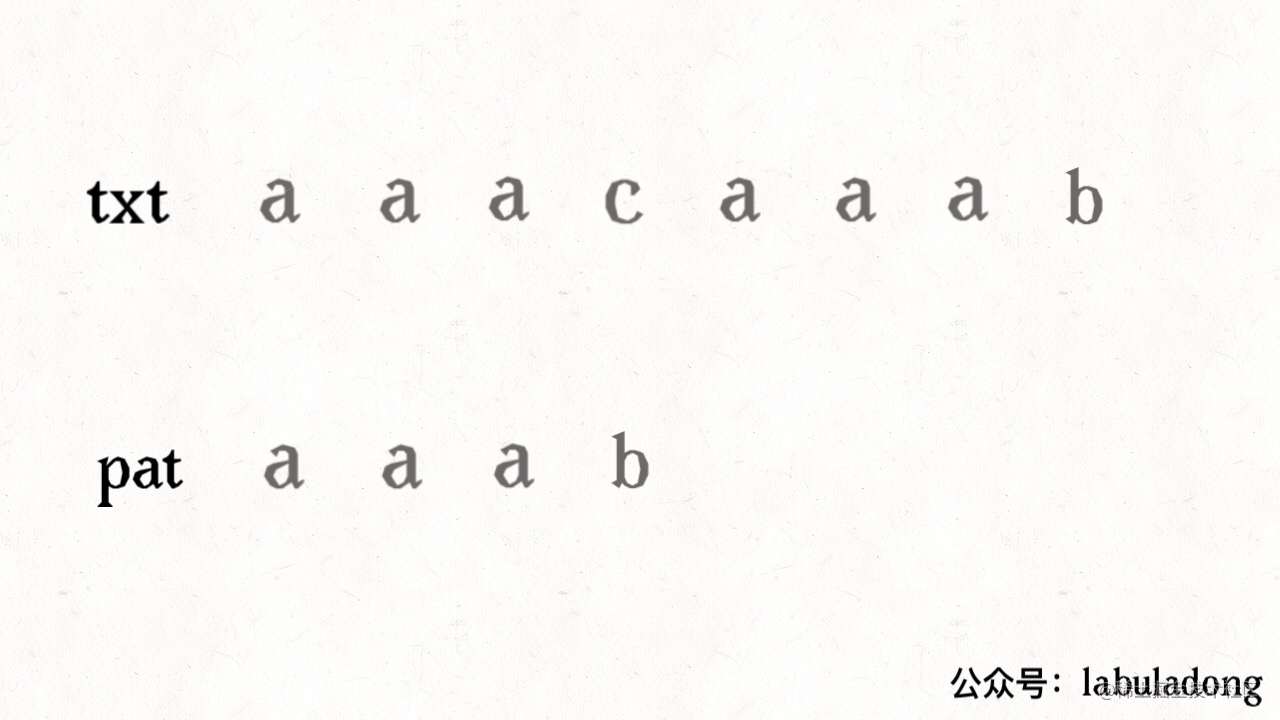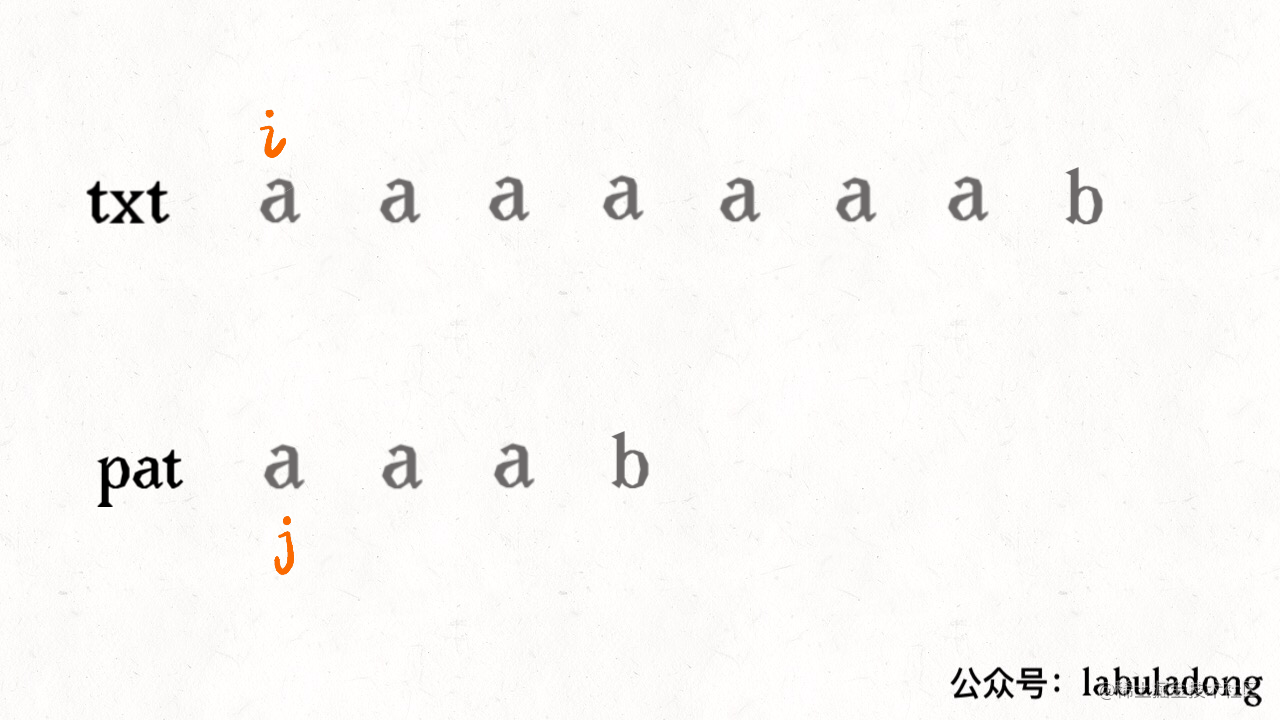KMP 算法永不回退 txt 的指针 i，不走回头路（不会重复扫描 txt），而是借助 dp 数组中储存的信息把 pat 移到正确的位置继续匹配，时间复杂度只需 O(N)，用空间换时间，所以我认为它是一种动态规划算法。

KMP 算法的难点在于，如何计算 dp 数组中的信息？如何根据这些信息正确地移动 pat 的指针？这个就需要确定有限状态自动机来辅助了，别怕这种高大上的文学词汇，其实和动态规划的 dp 数组如出一辙，等你学会了也可以拿这个词去吓唬别人。

txt1 = "aaacaaab"
pat = "aaab"
txt2 = "aaaaaaab"
pat = "aaab"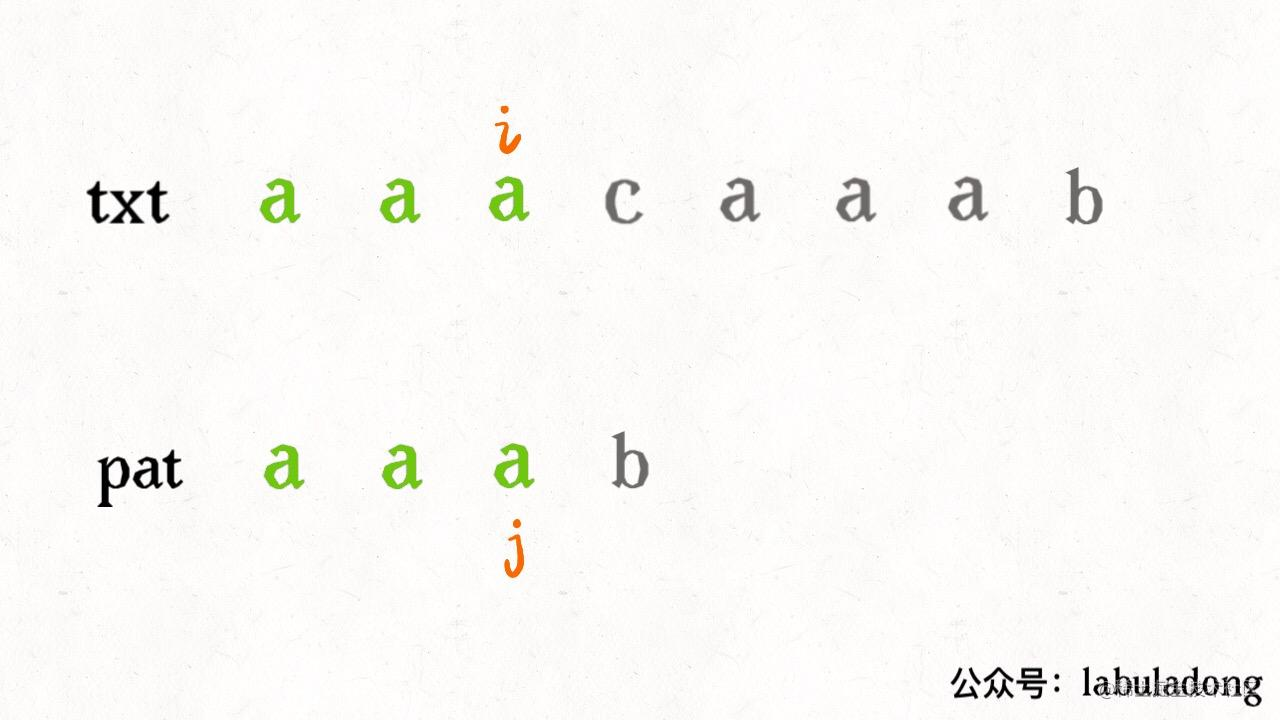dp 数组指示 pat 这样移动：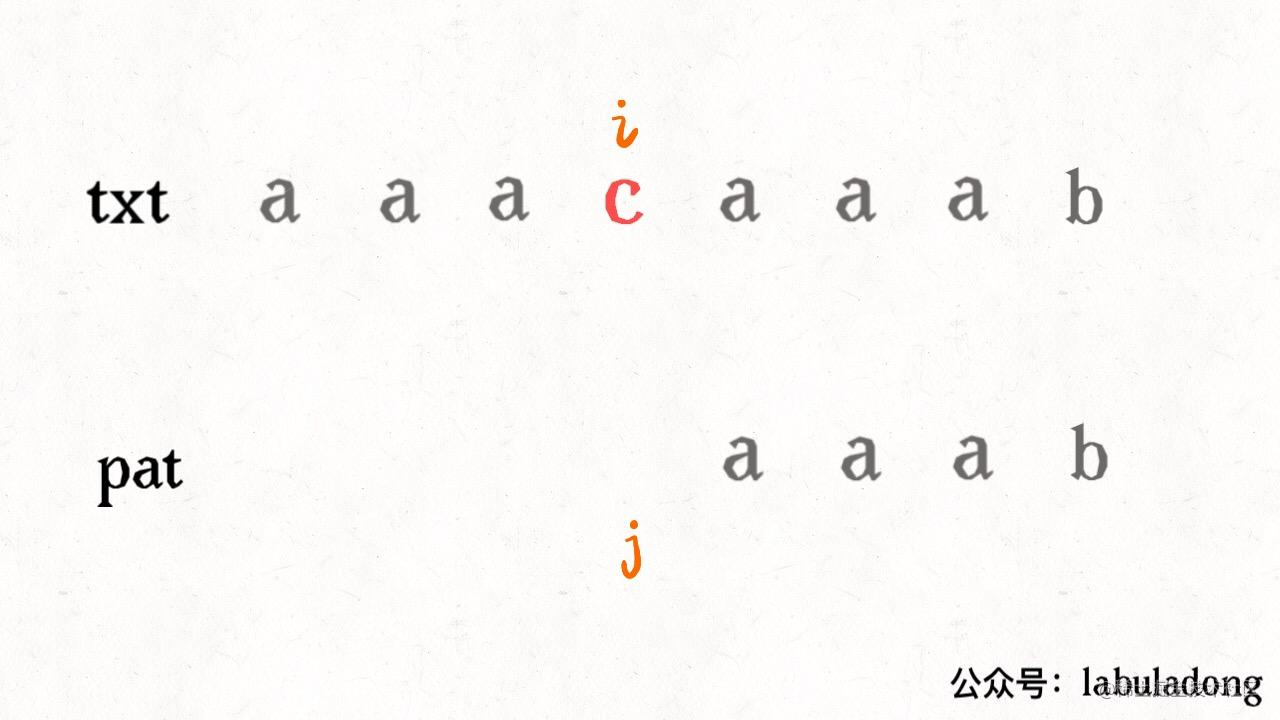PS：这个j 不要理解为索引，它的含义更准确地说应该是状态（state），所以它会出现这个奇怪的位置，后文会详述。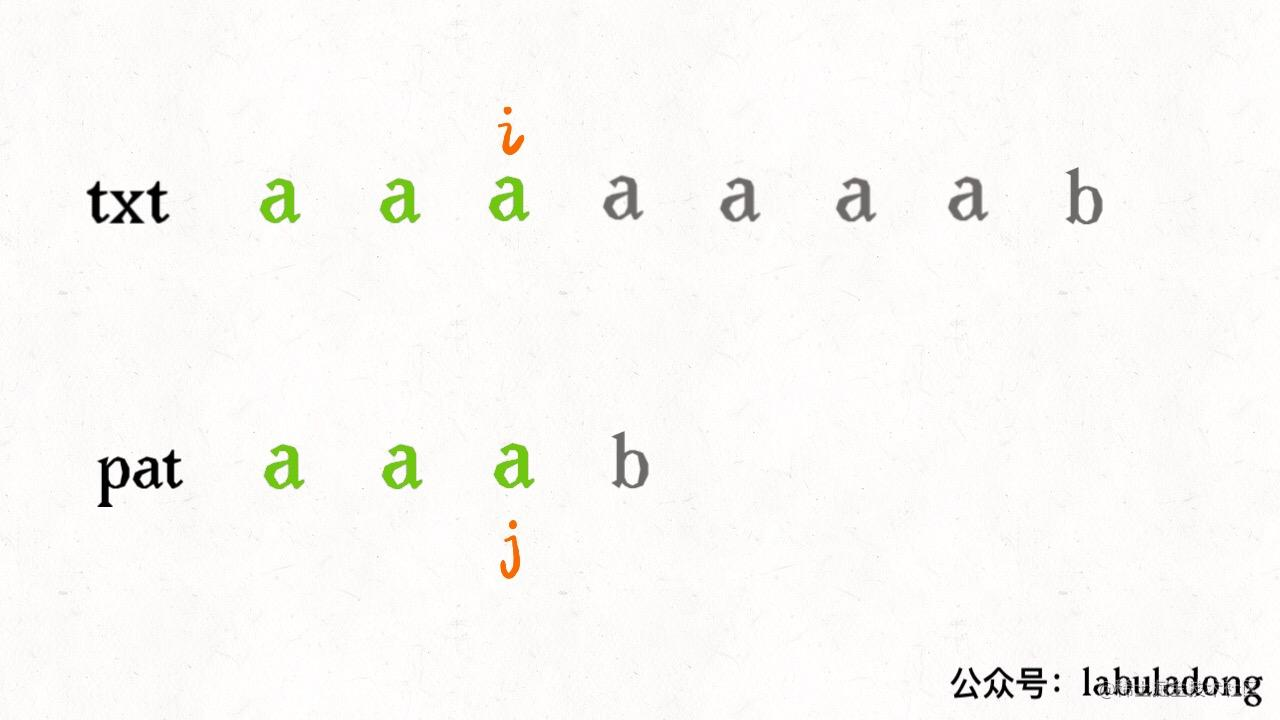dp 数组指示 pat 这样移动：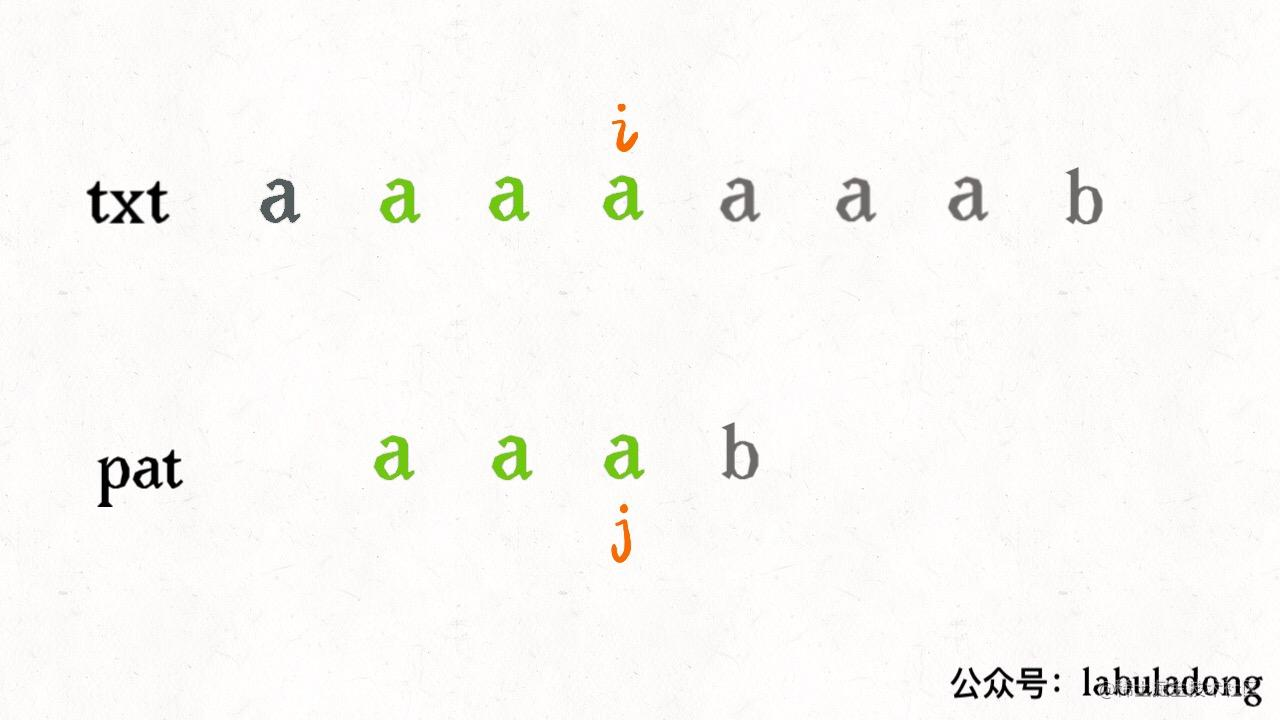public class KMP {
private int[][] dp;
private String pat;

public KMP(String pat) {
this.pat = pat;
// 通过 pat 构建 dp 数组
// 需要 O(M) 时间
}

public int search(String txt) {
// 借助 dp 数组去匹配 txt
// 需要 O(N) 时间
}
}

KMP kmp = new KMP("aaab");
int pos1 = kmp.search("aaacaaab"); //4
int pos2 = kmp.search("aaaaaaab"); //4

### 二、状态机概述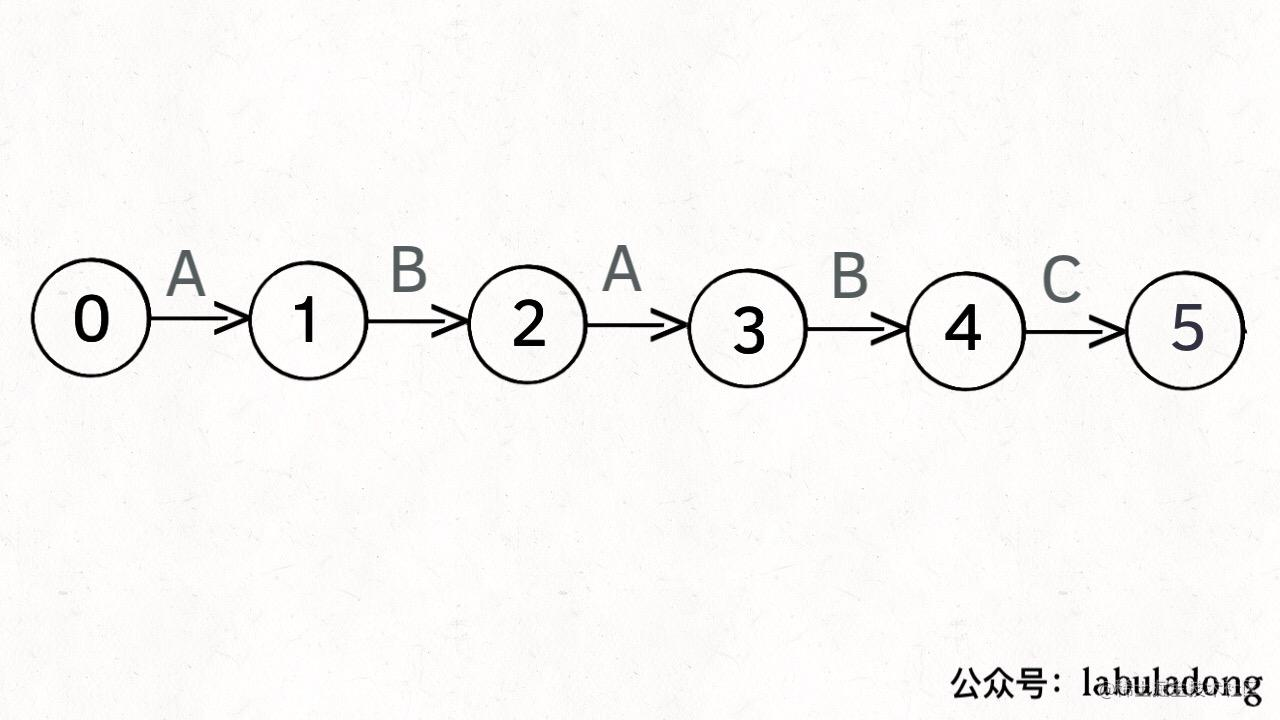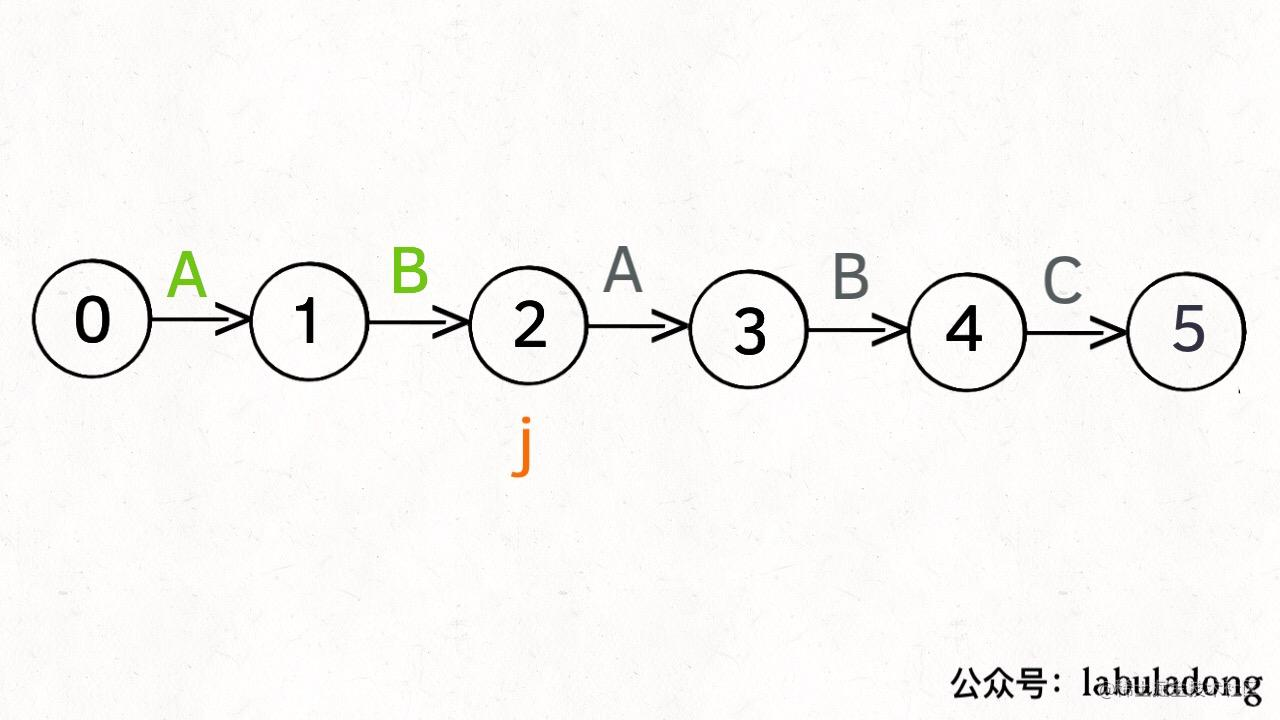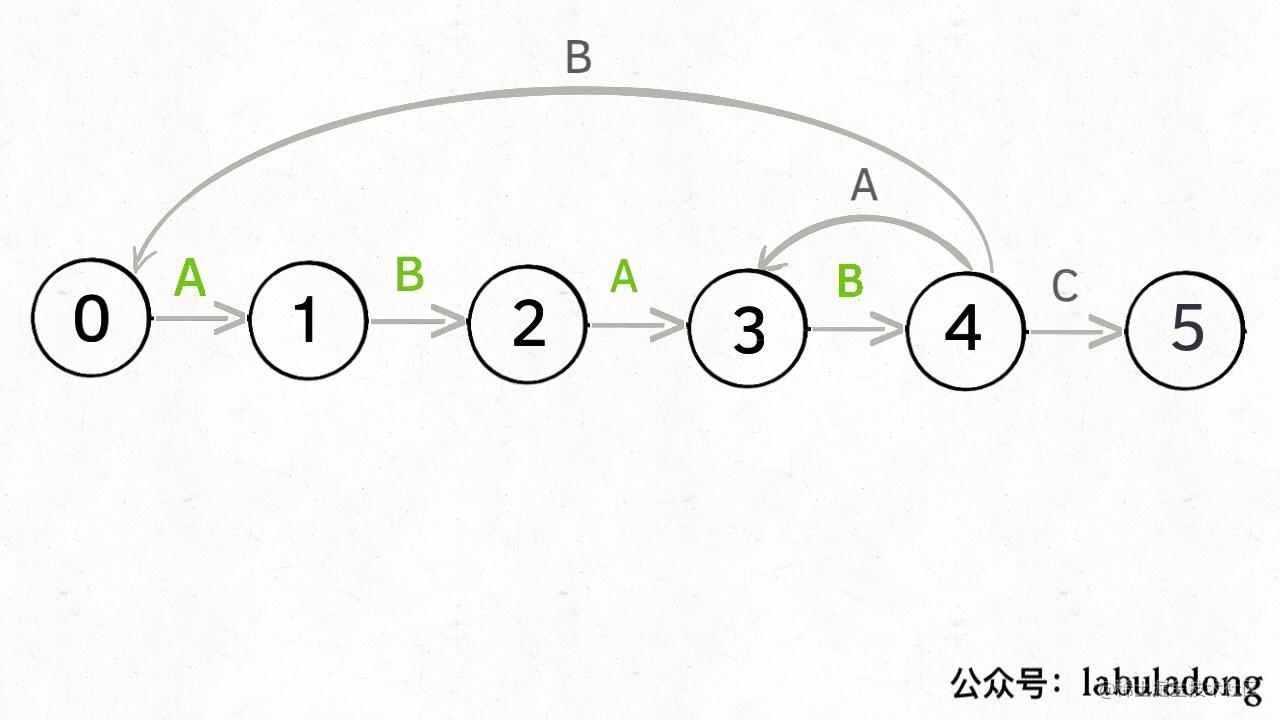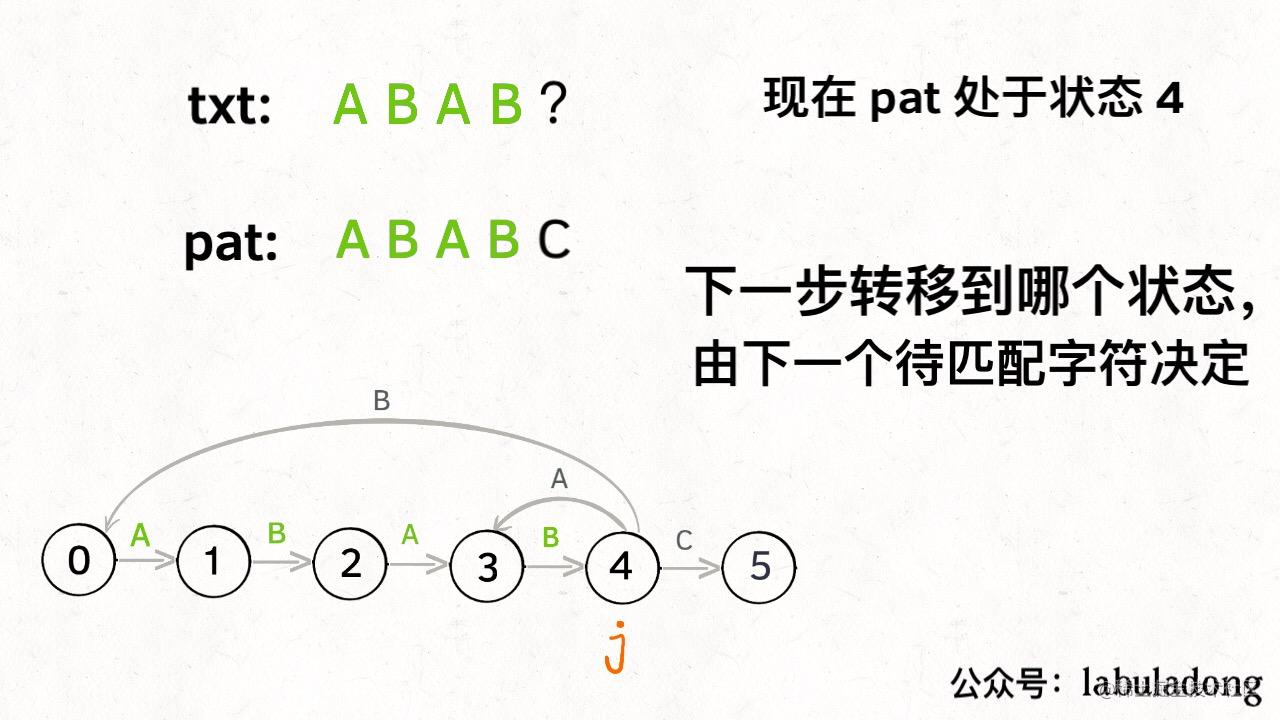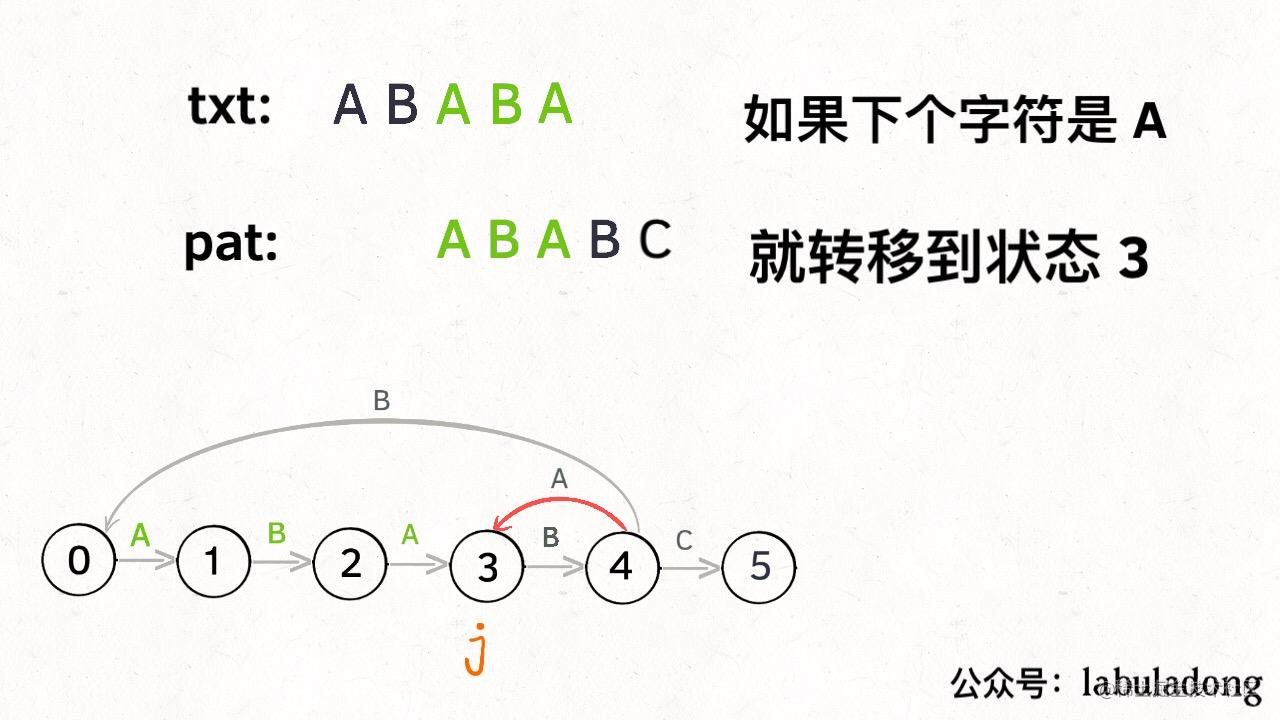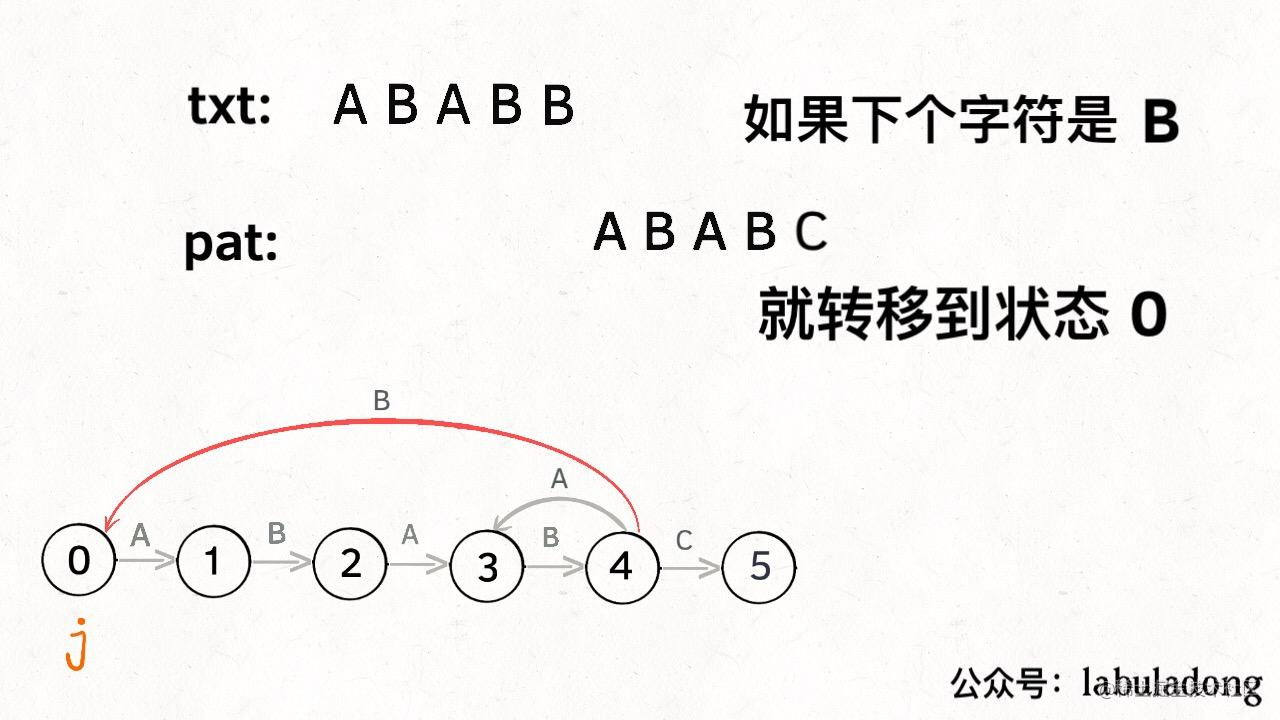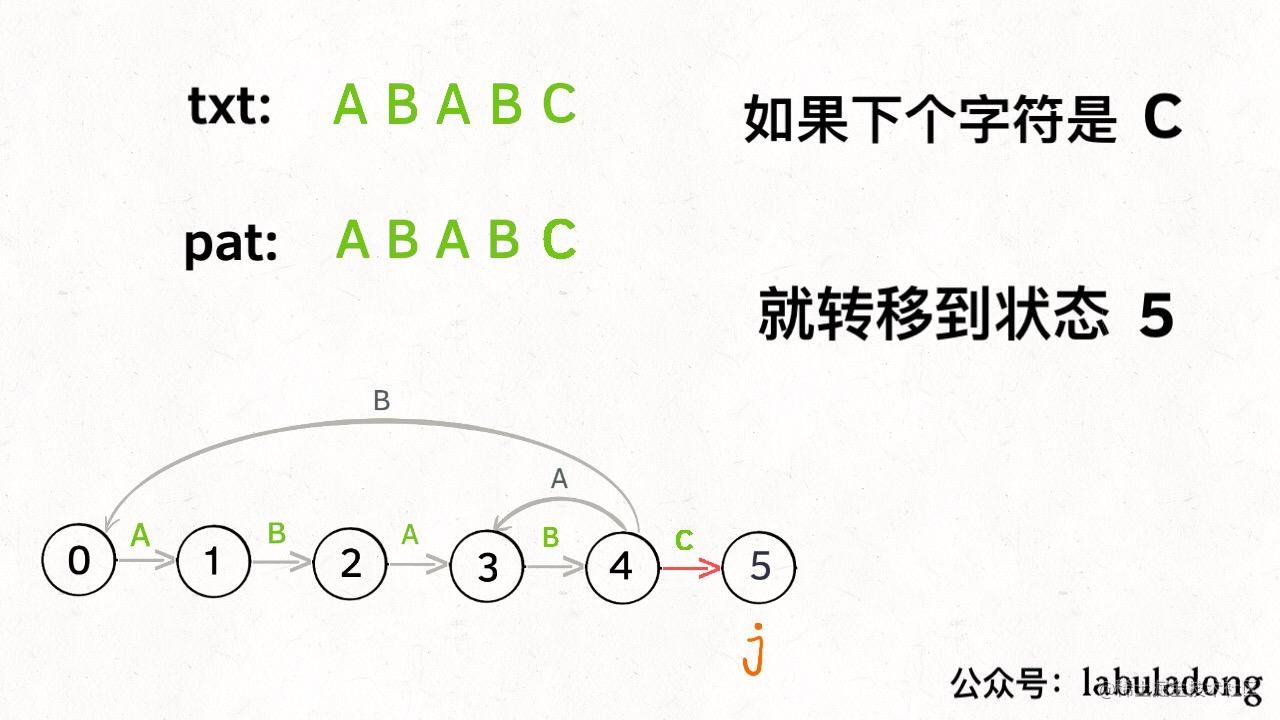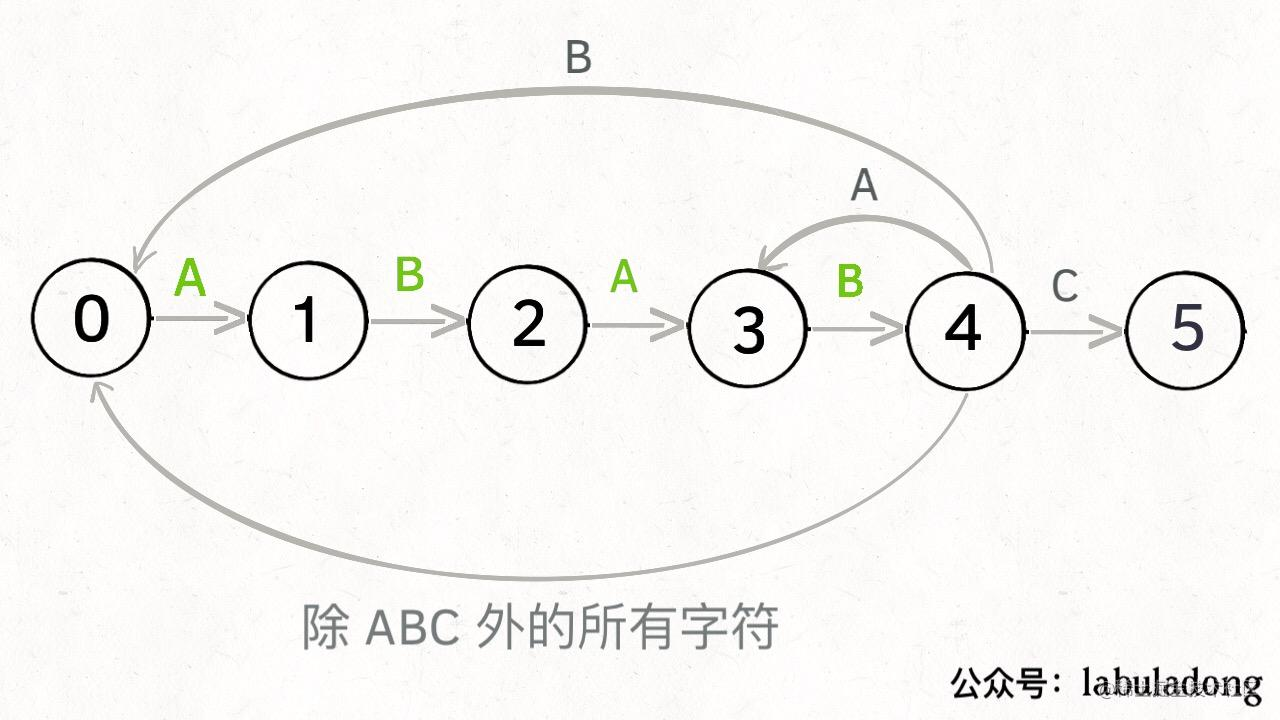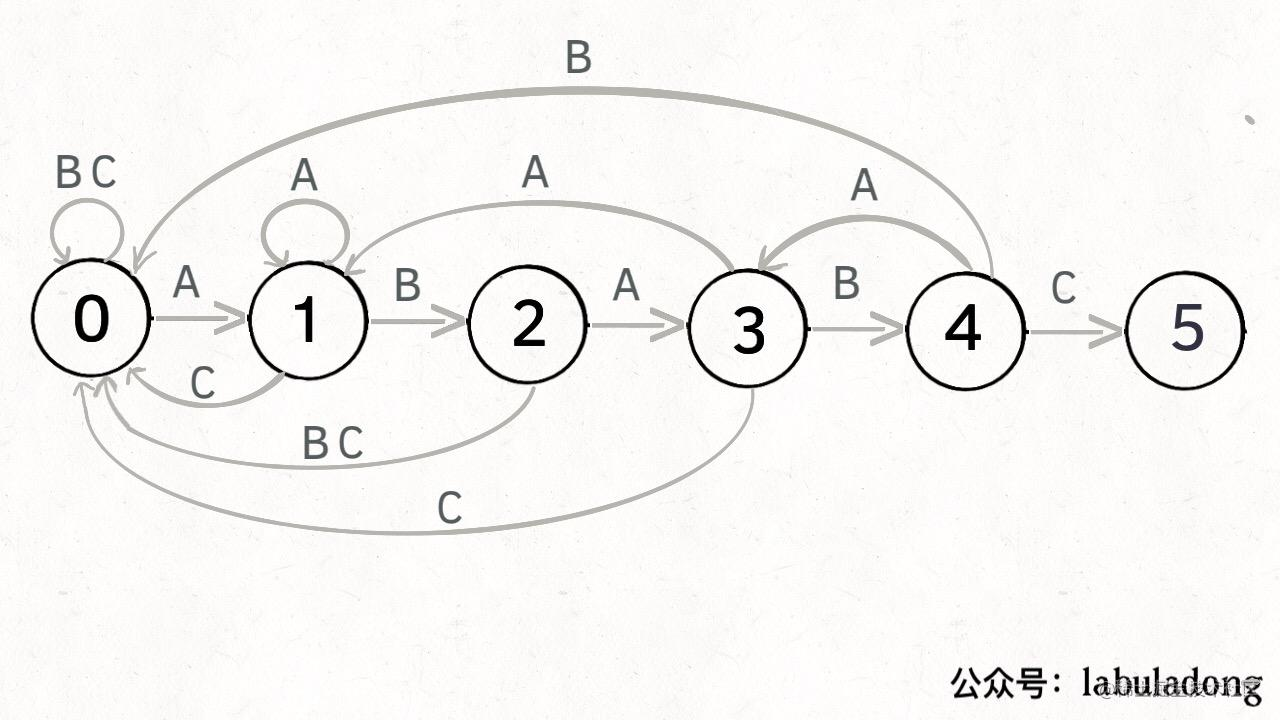KMP 算法最关键的步骤就是构造这个状态转移图。要确定状态转移的行为，得明确两个变量，一个是当前的匹配状态，另一个是遇到的字符；确定了这两个变量后，就可以知道这个情况下应该转移到哪个状态。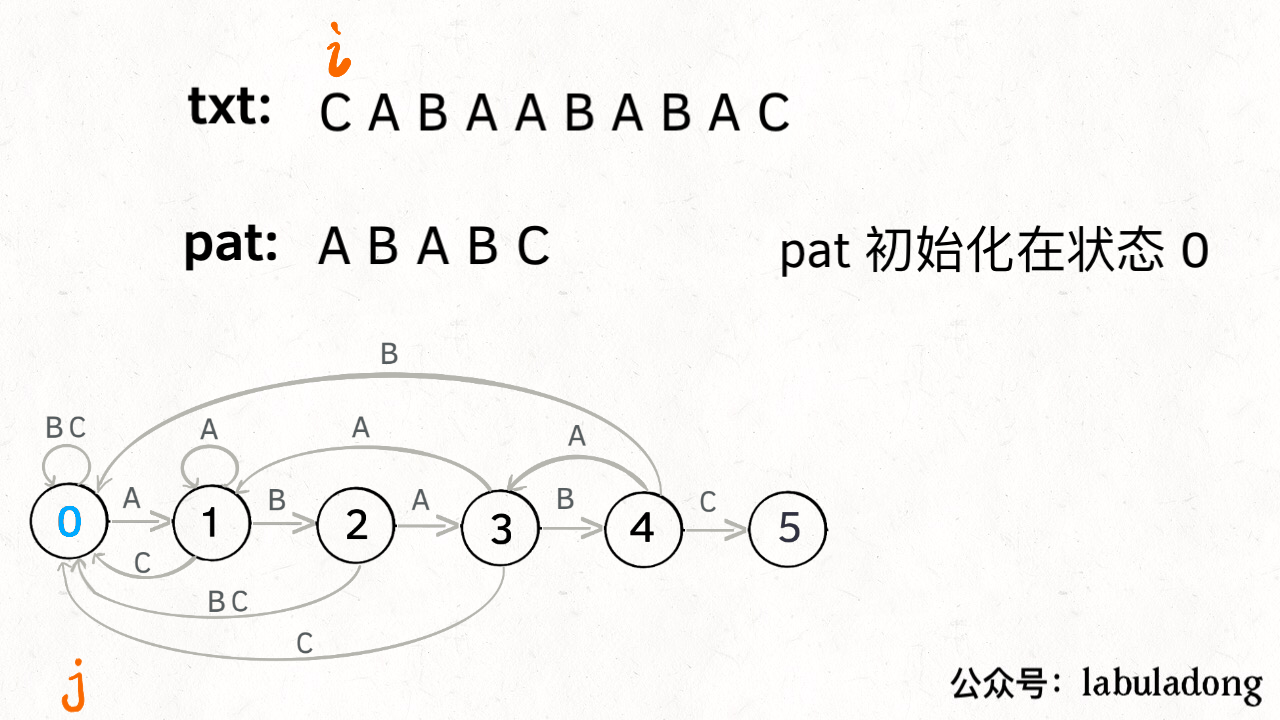dp[j][c] = next
0 <= j < M，代表当前的状态
0 <= c < 256，代表遇到的字符（ASCII 码）
0 <= next <= M，代表下一个状态

dp['A'] = 3 表示：

pat 应该转移到状态 3

dp['B'] = 2 表示：

pat 应该转移到状态 2

public int search(String txt) {
int M = pat.length();
int N = txt.length();
// pat 的初始态为 0
int j = 0;
for (int i = 0; i < N; i++) {
// 当前是状态 j，遇到字符 txt[i]，
// pat 应该转移到哪个状态？
j = dp[j][txt.charAt(i)];
// 如果达到终止态，返回匹配开头的索引
if (j == M) return i - M + 1;
}
// 没到达终止态，匹配失败
return -1;
}

### 三、构建状态转移图

for 0 <= j < M: # 状态
for 0 <= c < 256: # 字符
dp[j][c] = next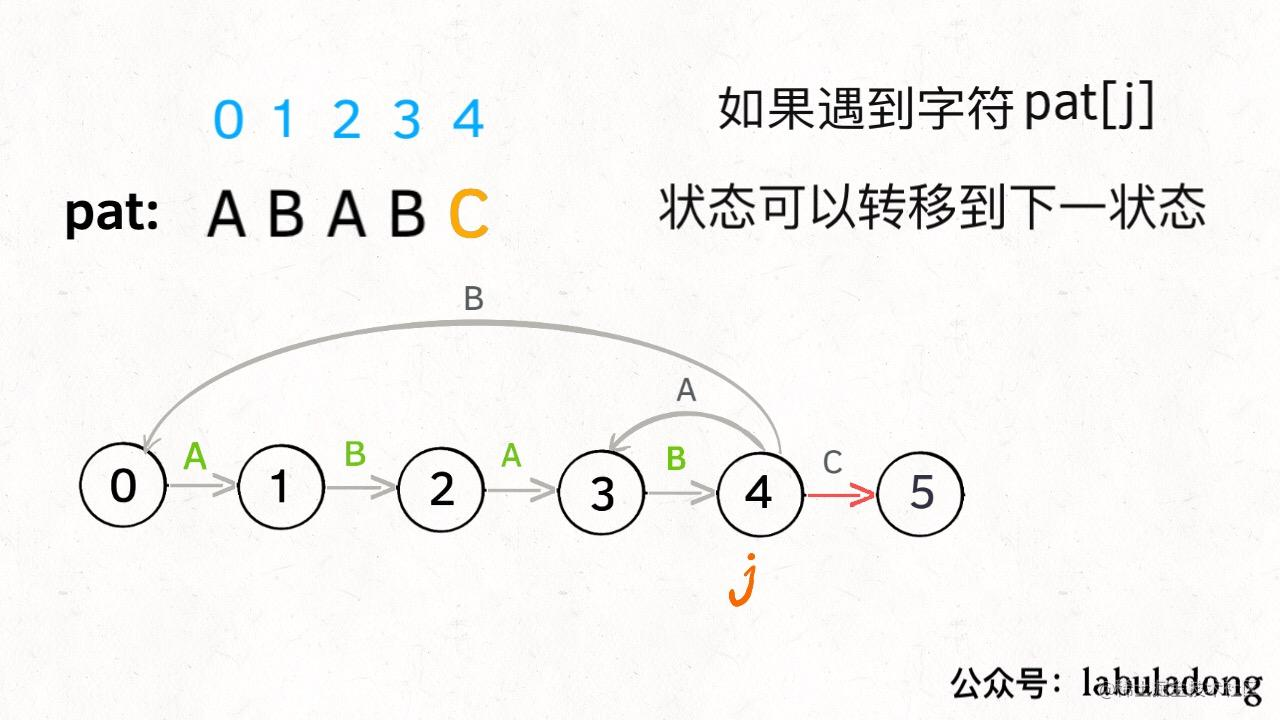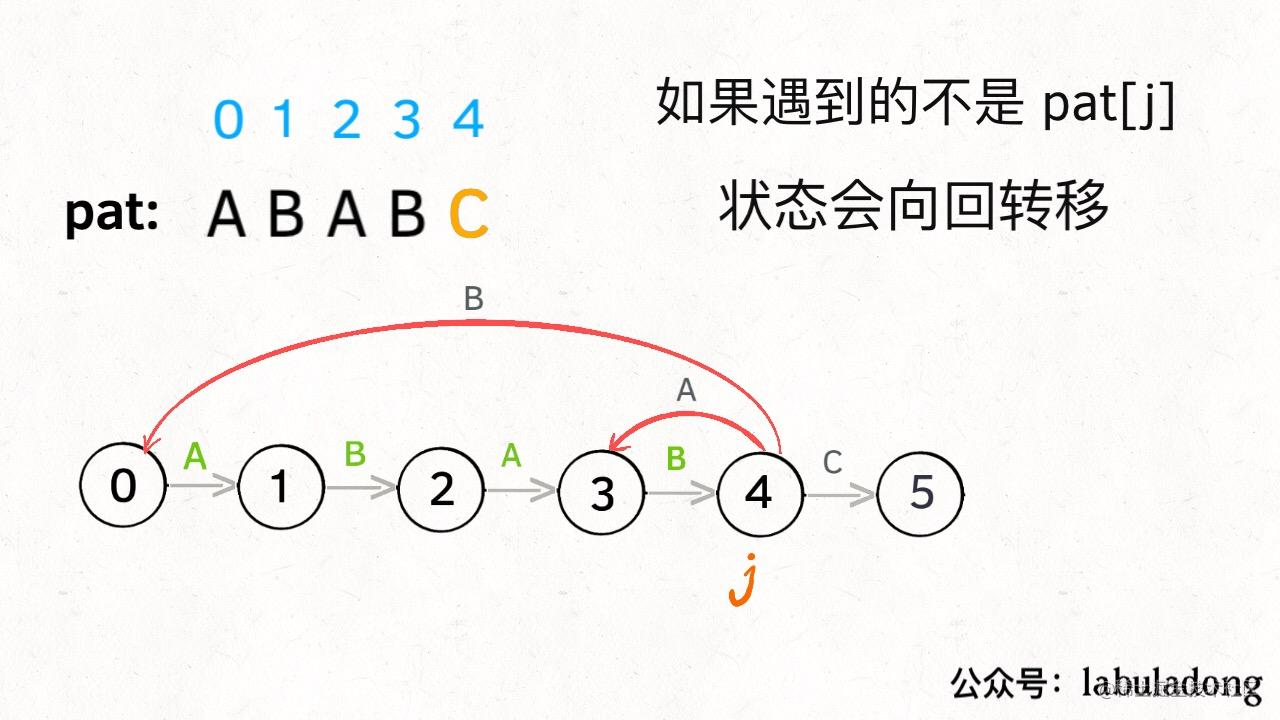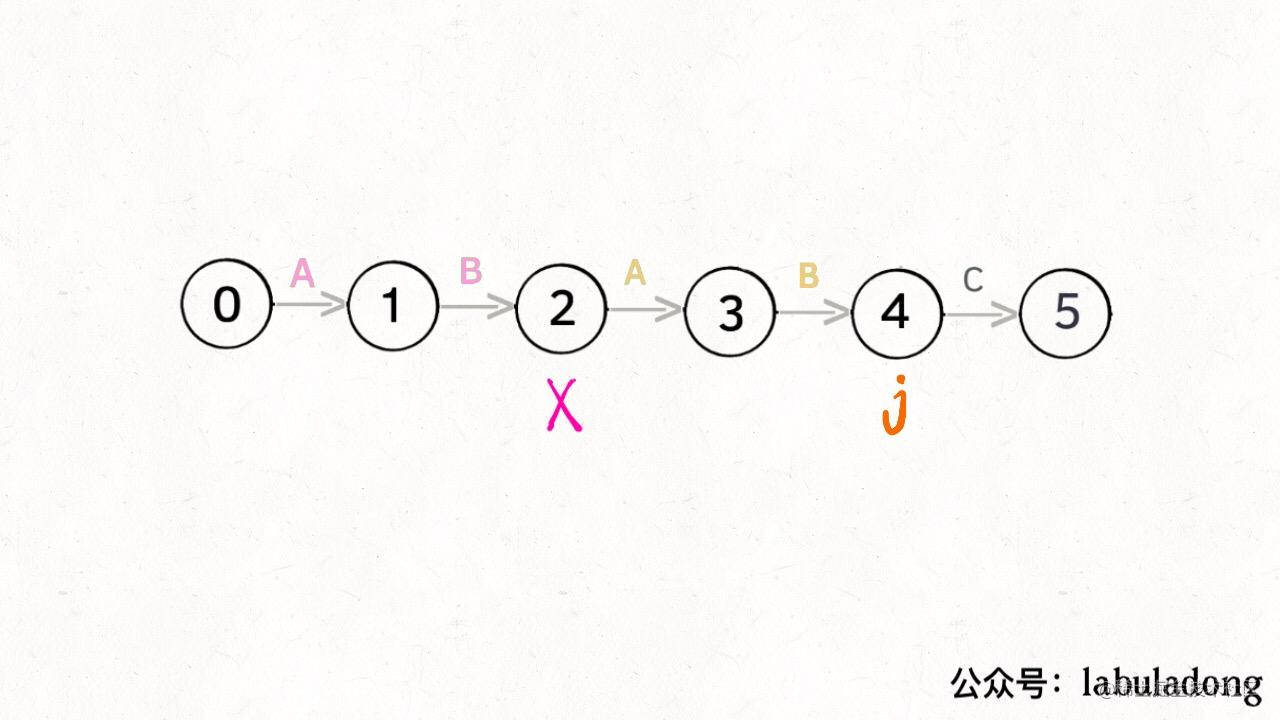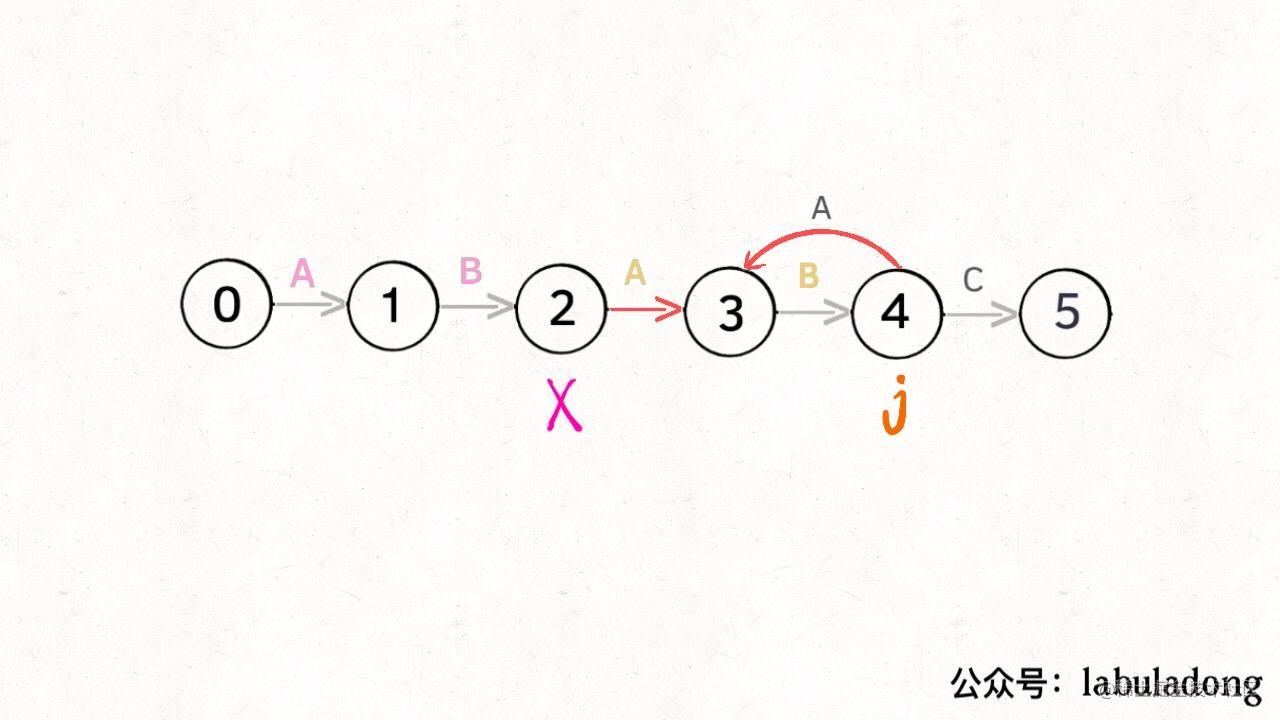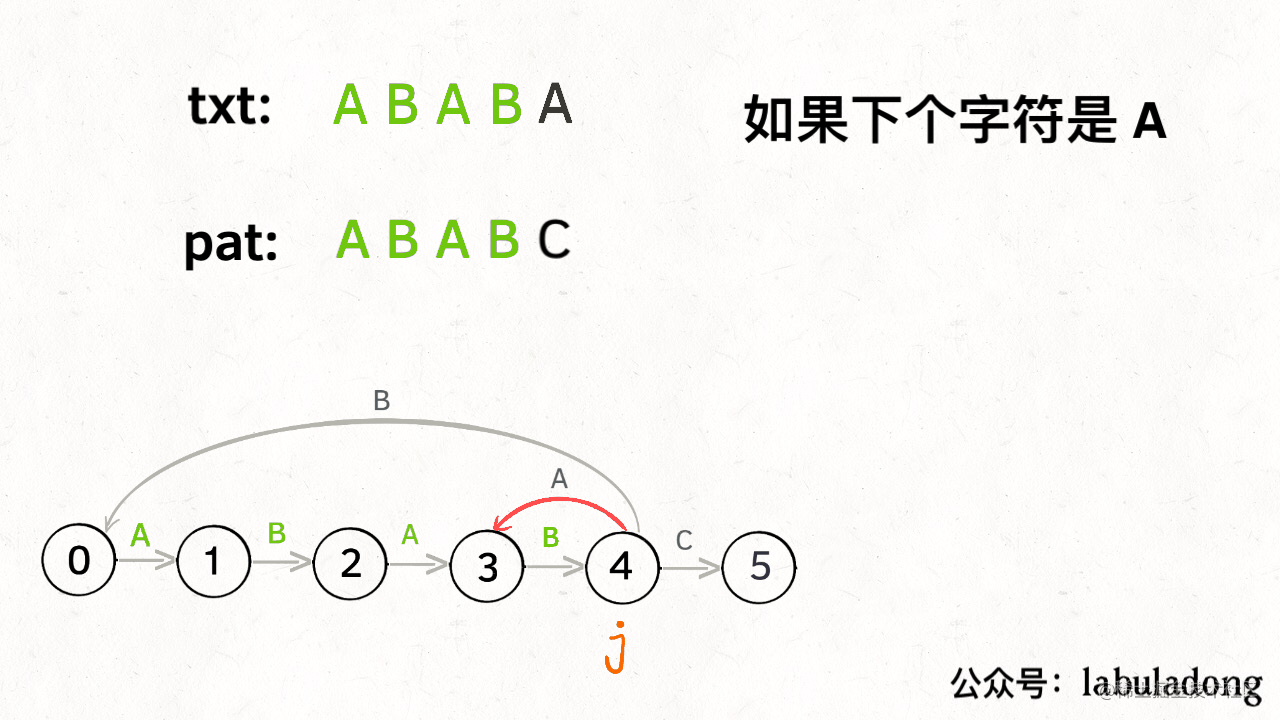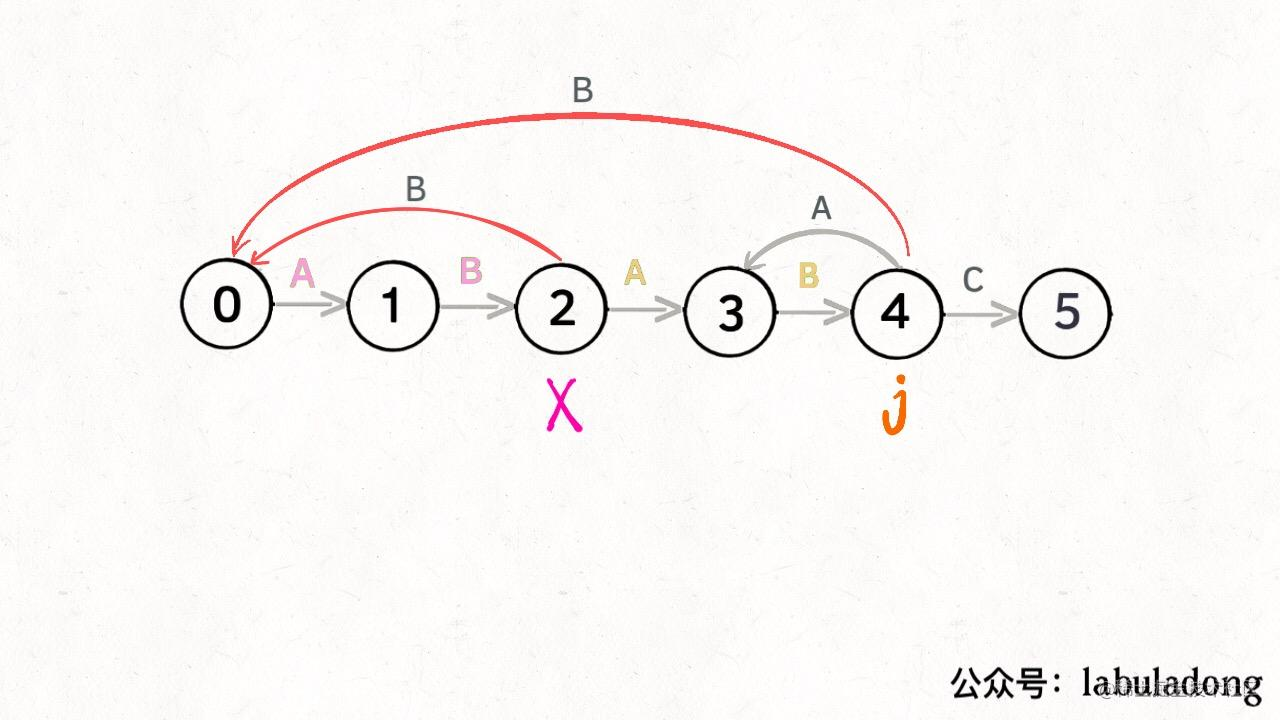int X # 影子状态
for 0 <= j < M:
for 0 <= c < 256:
if c == pat[j]:
# 状态推进
dp[j][c] = j + 1
else:
# 状态重启
# 委托 X 计算重启位置
dp[j][c] = dp[X][c]

### 四、代码实现

public class KMP {
private int[][] dp;
private String pat;

public KMP(String pat) {
this.pat = pat;
int M = pat.length();
// dp[状态][字符] = 下个状态
dp = new int[M];
// base case
dp[pat.charAt(0)] = 1;
// 影子状态 X 初始为 0
int X = 0;
// 当前状态 j 从 1 开始
for (int j = 1; j < M; j++) {
for (int c = 0; c < 256; c++) {
if (pat.charAt(j) == c)
dp[j][c] = j + 1;
else
dp[j][c] = dp[X][c];
}
// 更新影子状态
X = dp[X][pat.charAt(j)];
}
}

public int search(String txt) {...}
}

// base case
dp[pat.charAt(0)] = 1;

int X = 0;
for (int j = 1; j < M; j++) {
...
// 更新影子状态
// 当前是状态 X，遇到字符 pat[j]，
// pat 应该转移到哪个状态？
X = dp[X][pat.charAt(j)];
}

int j = 0;
for (int i = 0; i < N; i++) {
// 当前是状态 j，遇到字符 txt[i]，
// pat 应该转移到哪个状态？
j = dp[j][txt.charAt(i)];
...
}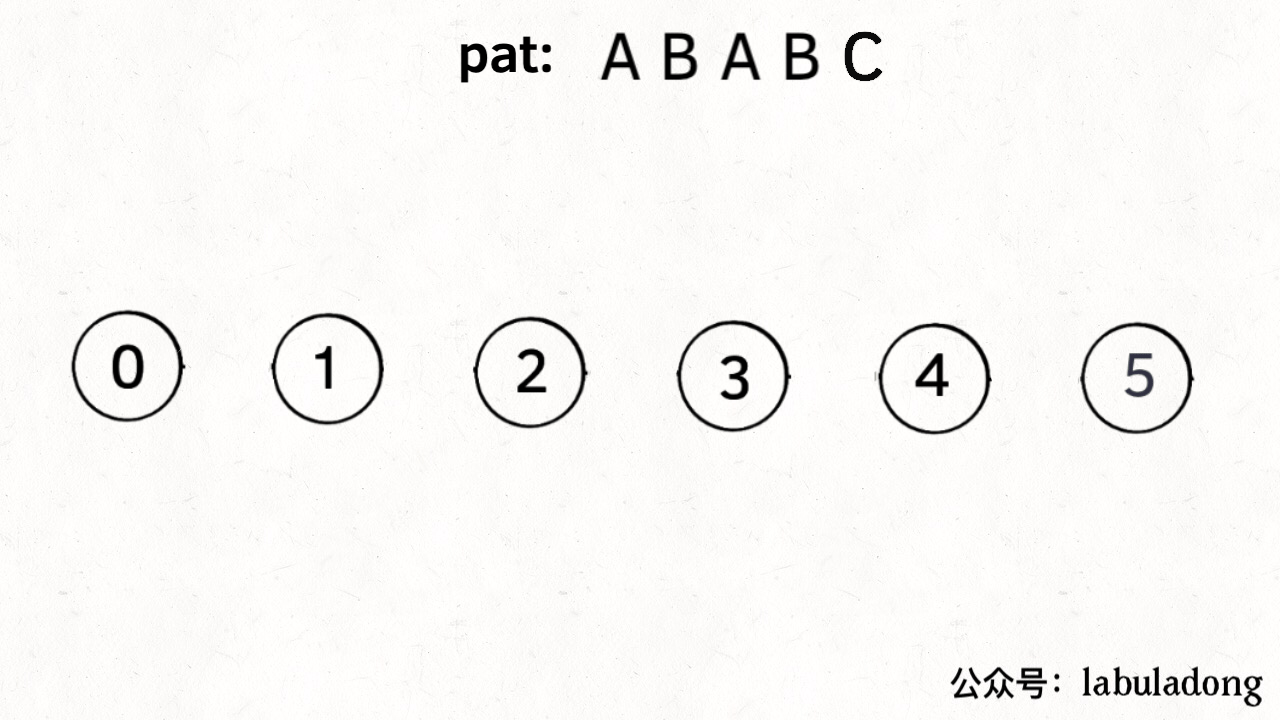public class KMP {
private int[][] dp;
private String pat;

public KMP(String pat) {
this.pat = pat;
int M = pat.length();
// dp[状态][字符] = 下个状态
dp = new int[M];
// base case
dp[pat.charAt(0)] = 1;
// 影子状态 X 初始为 0
int X = 0;
// 构建状态转移图（稍改的更紧凑了）
for (int j = 1; j < M; j++) {
for (int c = 0; c < 256; c++) {
dp[j][c] = dp[X][c];
dp[j][pat.charAt(j)] = j + 1;
// 更新影子状态
X = dp[X][pat.charAt(j)];
}
}

public int search(String txt) {
int M = pat.length();
int N = txt.length();
// pat 的初始态为 0
int j = 0;
for (int i = 0; i < N; i++) {
// 计算 pat 的下一个状态
j = dp[j][txt.charAt(i)];
// 到达终止态，返回结果
if (j == M) return i - M + 1;
}
// 没到达终止态，匹配失败
return -1;
}
}

### 五、最后总结

pat 匹配 txt 的过程中，只要明确了「当前处在哪个状态」和「遇到的字符是什么」这两个问题，就可以确定应该转移到哪个状态（推进或回退）。

dp[j][c] = next 表示，当前是状态 j，遇到了字符 c，应该转移到状态 next

KMP 算法也就是动态规划那点事，而且都是按照一套框架来的，无非就是描述问题逻辑，明确 dp 数组含义，定义 base case 这点破事。希望这篇文章能让大家对动态规划有更深的理解。

pat 匹配 txt 的过程中，只要明确了「当前处在哪个状态」和「遇到的字符是什么」这两个问题，就可以确定应该转移到哪个状态（推进或回退）。

dp[j][c] = next 表示，当前是状态 j，遇到了字符 c，应该转移到状态 next

KMP 算法也就是动态规划那点事，而且都是按照一套框架来的，无非就是描述问题逻辑，明确 dp 数组含义，定义 base case 这点破事。希望这篇文章能让大家对动态规划有更深的理解。